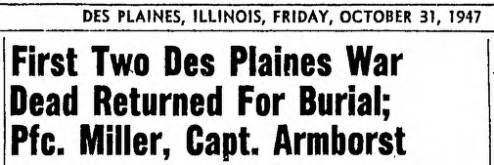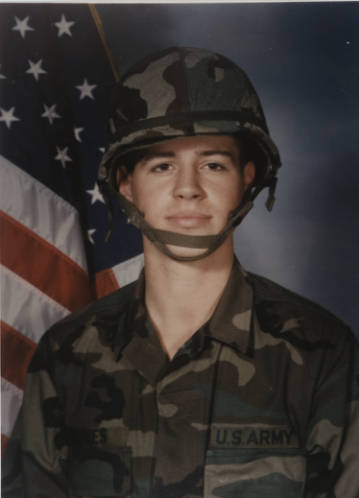Like   Tweet   Pin   +1   in

## November 2019

 table div table+table+table div table td,table.module-2{width:100%;padding:0}table div table+table+table div table{width:100%;float:none;margin-left:auto;margin-right:auto;padding:0}table div table+table+table div table a{border:0 none;text-decoration:none}table div table+table+table div table img{width:100%!important;border:0 none;text-decoration:none}/* styles */

## DES PLAINES HEROES: ON THE SEAS, IN THE AIR, AND ON FOREIGN SHORES

 /* styles */ On November 11, 1919, U.S. President Woodrow Wilson issued a statement declaring that November 11 shall be observed as Armistice Day, in honor of those Americans who had served in Europe during World War I. President Dwight D. Eisenhower changed Armistice Day to Veterans Day in 1954, broadening the scope of the holiday to include American veterans of all wars. In the Veterans Day Proclamation, Eisenhower wrote “…let us solemnly remember the sacrifices of all those who fought so valiantly, on the seas, in the air, and on foreign shores, to preserve our heritage of freedom, and let us reconsecrate ourselves to the task of promoting and enduring peace so that their efforts shall not have been in vain.”PFC Wilber C. Miller and Capt. Alfred H. Amborst were the first two World War II casualties from Des Plaines returned for burial, as reported by the Des Plaines Suburban Times.

Whether in times of war or times of peace, Des Plainesites have been serving in the military from the Civil War to the present day.

The 1861 diary of Adelia Thomas mentions several area boys who joined the Union Army after the battle of Fort Sumter. READ ADELIA’S DIARY IN OUR CIVIL WAR ERA COLLECTION HERE.

Below are just a few of the Des Plaines men and women who have served our country as represented in Des Plaines Memory. Many more can be found in the DPM Life During War collection.

## EXPLORE HERE

 table div table+table+table+table+table+table+table+table div table td,table.module-7{width:100%;padding:0}table div table+table+table+table+table+table+table+table div table{width:100%;float:none;margin-left:auto;margin-right:auto;padding:0}table div table+table+table+table+table+table+table+table div table a{border:0 none;text-decoration:none}table div table+table+table+table+table+table+table+table div table img{width:100%!important;border:0 none;text-decoration:none}/* styles */
 /* styles */ ABOVE: Clifton J. Hill served in the navy during World War I. This portrait of Hill in his uniform was most likely taken after the United States entered the war in April 1917.
 table div table+table+table+table+table+table+table+table+table+table div table td,table.module-9{width:100%;padding:0}table div table+table+table+table+table+table+table+table+table+table div table{width:100%;float:none;margin-left:auto;margin-right:auto;padding:0}table div table+table+table+table+table+table+table+table+table+table div table a{border:0 none;text-decoration:none}table div table+table+table+table+table+table+table+table+table+table div table img{width:100%!important;border:0 none;text-decoration:none}/* styles */
 /* styles */ ABOVE: Lieutenant Rose “Rosebud” A. Pieper served in the Army Nurse Corps during World War II. She graduated from the Walther School of Nursing in Chicago in May of 1944. The following spring, Pieper enlisted and was stationed at the Camp McCoy Station Hospital in Wisconsin. We see her here in uniform, circa 1945.
 table div table+table+table+table+table+table+table+table+table+table+table+table div table td,table.module-11{width:100%;padding:0}table div table+table+table+table+table+table+table+table+table+table+table+table div table{width:100%;float:none;margin-left:auto;margin-right:auto;padding:0}table div table+table+table+table+table+table+table+table+table+table+table+table div table a{border:0 none;text-decoration:none}table div table+table+table+table+table+table+table+table+table+table+table+table div table img{width:100%!important;border:0 none;text-decoration:none}/* styles */
 table.module-12{width:99.81%;padding:0}table div table+table+table+table+table+table+table+table+table+table+table+table+table div table{width:99.81%;float:none;margin-left:auto;margin-right:auto;padding:0}table div table+table+table+table+table+table+table+table+table+table+table+table+table div table a{border:0 none;text-decoration:none}table div table+table+table+table+table+table+table+table+table+table+table+table+table div table img{width:100%!important;border:0 none;text-decoration:none}table div table+table+table+table+table+table+table+table+table+table+table+table+table div table td{width:100%;padding:0}/* styles */
 /* styles */ ABOVE: Betty Jo Streff, a Douglas Aircraft employee and aviation buff, made history as one of the original Women's Airforce Service Pilots (WASP). She enlisted in February 1944 and served at Columbus Army Air Field, Columbus, Mississippi, where she flew Beechcraft AT-10’s and BT-13’s as an engineering test pilot, ferry pilot and administrative pilot. She and her fellow WASP were awarded the Congressional Gold Medal in 2010 in recognition of their service.
 table div table+table+table+table+table+table+table+table+table+table+table+table+table+table+table div table td,table.module-14{width:100%;padding:0}table div table+table+table+table+table+table+table+table+table+table+table+table+table+table+table div table{width:100%;float:none;margin-left:auto;margin-right:auto;padding:0}table div table+table+table+table+table+table+table+table+table+table+table+table+table+table+table div table a{border:0 none;text-decoration:none}table div table+table+table+table+table+table+table+table+table+table+table+table+table+table+table div table img{width:100%!important;border:0 none;text-decoration:none}/* styles */
 /* styles */ ABOVE: Marvin M. Kingdon (Sgt., USMC), one of many Des Plaines citizens who served during the Korean war, was awarded the Purple Heart medal, as seen here in the October 30, 1952 issue of the Des Plaines Suburban Times.ABOVE: In more recent times, Des Plaines resident Brian D. Jones served during Operation Desert Storm, 1991. This photo was part of a project by the Des Plaines Historical Society to collect images of local service members in that conflict.

## THERE'S MORE! EXPLORE THE DES PLAINES VETERANS COLLECTION HERE

 table div table+table+table+table+table+table+table+table+table+table+table+table+table+table+table+table+table+table+table div table{width:100%;padding:0}table div table+table+table+table+table+table+table+table+table+table+table+table+table+table+table+table+table+table+table div table img{width:96.23%;padding:0;float:none}table div table+table+table+table+table+table+table+table+table+table+table+table+table+table+table+table+table+table+table div table td{width:100%;padding:0 1.88% 18px}/* styles */Are you now or have you ever served in any branch of the U.S. military, or do you know someone that is currently or has ever served in the military?

## PLEASE UPLOAD YOUR PHOTOGRAPHS DIRECTLY TO DES PLAINES MEMORY HERE AND BECOME A PART OF THIS UNIQUE LIBRARY COLLECTION/* styles */ ABOVE: American Legion Post 36, Operation Show Your Colors. Photograph of American Legionnaires who were passing out American flag lapel pins at the Des Plaines railroad station to mark Pearl Harbor Day. From the February 14, 1965 edition of the Des Plaines Suburban Times.
 table div table+table+table+table+table+table+table+table+table+table+table+table+table+table+table+table+table+table+table+table+table+table+table+table div table{width:100%;padding:0}table div table+table+table+table+table+table+table+table+table+table+table+table+table+table+table+table+table+table+table+table+table+table+table+table div table img{width:96.23%;padding:0;float:none}table div table+table+table+table+table+table+table+table+table+table+table+table+table+table+table+table+table+table+table+table+table+table+table+table div table td{width:100%;padding:0 1.88% 18px}/* styles */## Library Resources/* styles */ Fold3 is a convenient access to US military records, including the stories, photos, and personal documents of the men and women who served. Historical documents like the National Archives Holocaust Records are also included. A useful tool for historians, genealogists, researchers, military enthusiasts, veterans and their families and teachers. CHECK IT OUT
 table div table+table+table+table+table+table+table+table+table+table+table+table+table+table+table+table+table+table+table+table+table+table+table+table+table+table+table+table div table{width:100%;padding:0}table div table+table+table+table+table+table+table+table+table+table+table+table+table+table+table+table+table+table+table+table+table+table+table+table+table+table+table+table div table img{width:96.23%;padding:0;float:none}table div table+table+table+table+table+table+table+table+table+table+table+table+table+table+table+table+table+table+table+table+table+table+table+table+table+table+table+table div table td{width:100%;padding:0 1.88% 18px}/* styles */## Upcoming Programs

 table div table+table+table+table+table+table+table+table+table+table+table+table+table+table+table+table+table+table+table+table+table+table+table+table+table+table+table+table+table+table div table td,table.module-29{width:100%;padding:0}table div table+table+table+table+table+table+table+table+table+table+table+table+table+table+table+table+table+table+table+table+table+table+table+table+table+table+table+table+table+table div table{width:100%;float:none;margin-left:auto;margin-right:auto;padding:0}table div table+table+table+table+table+table+table+table+table+table+table+table+table+table+table+table+table+table+table+table+table+table+table+table+table+table+table+table+table+table div table a{border:0 none;text-decoration:none}table div table+table+table+table+table+table+table+table+table+table+table+table+table+table+table+table+table+table+table+table+table+table+table+table+table+table+table+table+table+table div table img{width:100%!important;border:0 none;text-decoration:none}/* styles */
 table div table+table+table+table+table+table+table+table+table+table+table+table+table+table+table+table+table+table+table+table+table+table+table+table+table+table+table+table+table+table+table div table td,table.module-30{width:100%;padding:0}table div table+table+table+table+table+table+table+table+table+table+table+table+table+table+table+table+table+table+table+table+table+table+table+table+table+table+table+table+table+table+table div table{width:100%;float:none;margin-left:auto;margin-right:auto;padding:0}table div table+table+table+table+table+table+table+table+table+table+table+table+table+table+table+table+table+table+table+table+table+table+table+table+table+table+table+table+table+table+table div table a{border:0 none;text-decoration:none}table div table+table+table+table+table+table+table+table+table+table+table+table+table+table+table+table+table+table+table+table+table+table+table+table+table+table+table+table+table+table+table div table img{width:100%!important;border:0 none;text-decoration:none}/* styles */
 table div table+table+table+table+table+table+table+table+table+table+table+table+table+table+table+table+table+table+table+table+table+table+table+table+table+table+table+table+table+table+table+table div table{width:100%;padding:0}table div table+table+table+table+table+table+table+table+table+table+table+table+table+table+table+table+table+table+table+table+table+table+table+table+table+table+table+table+table+table+table+table div table img{width:96.23%;padding:0;float:none}table div table+table+table+table+table+table+table+table+table+table+table+table+table+table+table+table+table+table+table+table+table+table+table+table+table+table+table+table+table+table+table+table div table td{width:100%;padding:0 1.88% 18px}/* styles */Steven Giese, Des Plaines Memory Curator